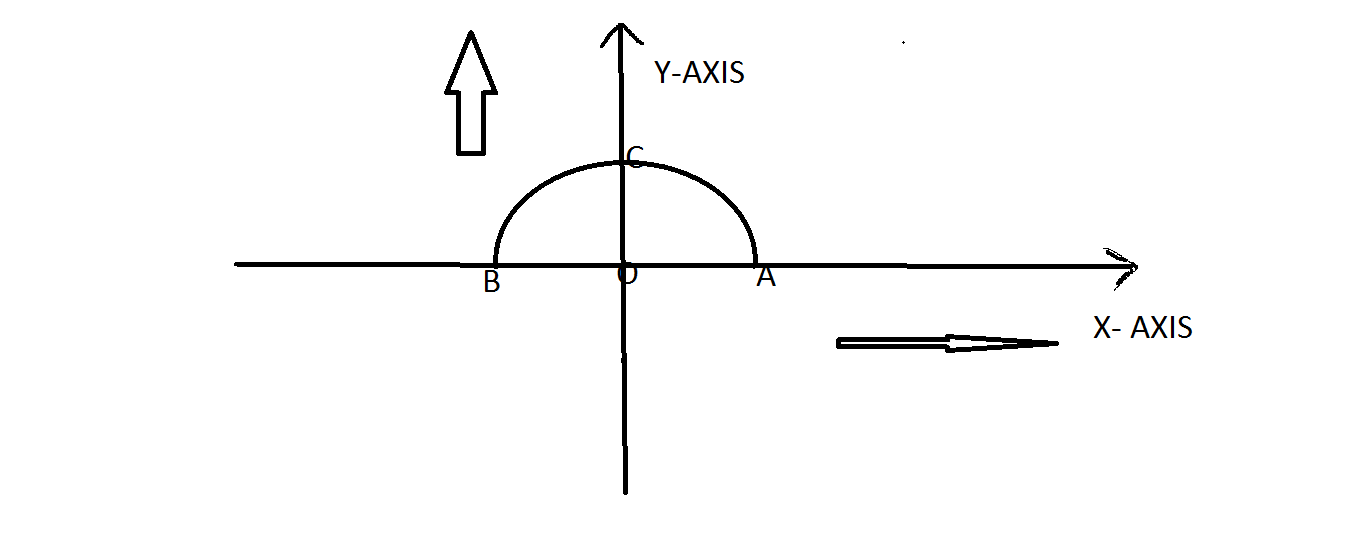# Parabola

How high is a parabolic arch of span 24 m and height 18m at a distance of 8 m from the centre of the span?

•Amartya Kumar Mistry ·

TAKE ORIGIN AS THE CENTER OF THE SPAN NOW FROM THE PROBLEM AB=24 AND OC=18 SO FROM CO-ORDINATE CONCEPT YOU WILL GET A(12,0) AND B(-12,0). NOW ASSUME THAT IT IS A PARABOLA OF TYPE X^2=4AY THE PARABOLA HAS VERTEX C(0,18)

NOW THE EQUATION BECOMES X^2=4a(Y-18)
AS THE PARABOLA PASSES THROUGH A IT WILL SATISFY THE PARABOLA EQ FROM THERE YOU WILL GET A=-2
TO GET YOUR ANSWER PUT X=8 AND YOU WILL GET HEIGHT 10 m

•Amartya Kumar Mistry ·•